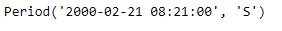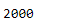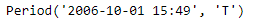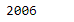Open in App
Not now

# Python | Pandas Period.year

• Last Updated : 06 Jan, 2019

Python is a great language for doing data analysis, primarily because of the fantastic ecosystem of data-centric python packages. Pandas is one of those packages and makes importing and analyzing data much easier.

Pandas` Period.year` attribute return an integer value representing the year the given period lies in.

Syntax : Period.year

Parameters : None

Return : year

Example #1: Use `Period.year `attribute to find the year the period lies in for the given Period object.

 `# importing pandas as pd``import` `pandas as pd`` ` `# Create the Period object``prd ``=` `pd.Period(freq ``=``'S'``, year ``=` `2000``, month ``=` `2``,``                  ``day ``=` `21``, hour ``=` `8``, minute ``=` `21``)`` ` `# Print the Period object``print``(prd)`

Output :Now we will use the `Period.year` attribute to find the value of year

 `# return the year value``prd.year`

Output :As we can see in the output, the `Period.year `attribute has returned 2000 indicating that the given period lies in the year of 2000.

Example #2: Use `Period.year` attribute to find the year the period lies in for the given Period object.

 `# importing pandas as pd``import` `pandas as pd`` ` `# Create the Period object``prd ``=` `pd.Period(freq ``=``'T'``, year ``=` `2006``, month ``=` `10``,``                            ``hour ``=` `15``, minute ``=` `49``)`` ` `# Print the Period object``print``(prd)`

Output :Now we will use the `Period.year` attribute to find the value of year

 `# return the year``prd.year`

Output :As we can see in the output, the `Period.year `attribute has returned 2006 indicating that the given period lies in the year of 2006.

My Personal Notes arrow_drop_up